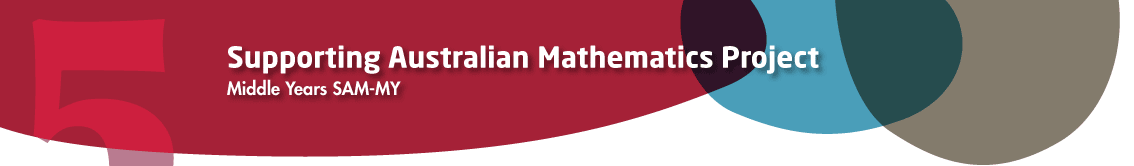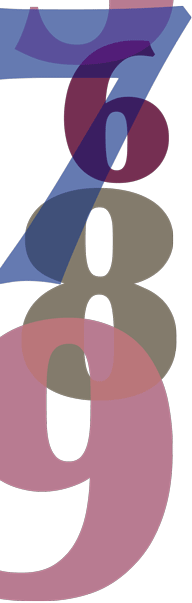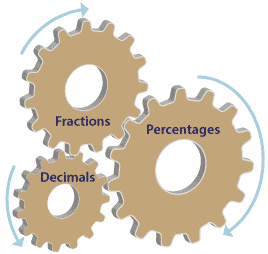# Year 6

## Number and Algebra

### Connecting fractions, decimals and percentagesDetailed description

There is a relationship between decimals, fractions and percentages. Whilst each of these areas is often taught separately, there is value in demonstrating how fractions, decimals and percentages are related.

Decimals are a convenient and useful way of writing fractions with denominators 10, 100, 1000 and so on.

So $$\dfrac{3}{10}$$ is written as 0.3, $$\dfrac{2}{100}$$ is written as 0.02, $$\dfrac{11}{100}$$ is written as 0.11 and we write $$\dfrac{434}{1000}$$ as 0.434 in decimal form.

This is best done with simple, familiar fractions, decimals and percentages to start with.Detailed description

Even though there are different ways of writing a number, the value remains the same.